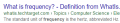# Period and Aliasing Problems

#### josh007

Joined Sep 20, 2015
43
Good day All,

I need your valued precious advice and help once again, in correcting my tutorial homework examples.

Please can you check my work if I am correct or advice where I am going wrong.

gracias

Q1)

If the signal x(t)=4cos(200πt) is sampled at 500 Hz, determine the signal x(n).

What is the period of the sampled signal?

x(t)=4cos((200/500)πt)

so my signal x(n) = 4cos((2/5)πt)

P = 2π/w

Where w is the angular velocity = 2π/5

P = 2π/(2π/5) = 5 units

So Period is 5 units

Q2)

Determine the minimum sampling rate to avoid aliasing for the signal x(t)=20cos(400πt)+10cos(20πt).

I will take the highest frequency to avoid aliasing.

So P = 2π/w = 2π/400π = 1/200

Therefore f= 1/T = 1/(1/200) = 200hz

To avoid aliasing fx2 = 400hz

#### WBahn

Joined Mar 31, 2012
26,326
Looks good, except that sampling rates should not be given in Hz (though they often are), since 1 Hz is one cycle per second. Instead, the units should be Sa/s (samples per second).

•josh007

#### josh007

Joined Sep 20, 2015
43
Looks good, except that sampling rates should not be given in Hz (though they often are), since 1 Hz is one cycle per second. Instead, the units should be Sa/s (samples per second).
Fantastic, much appreciation WBahn, I will take note of this. You are correct I read in my text book about the Sa/s but completely forgot about it.

Gracias

#### crutschow

Joined Mar 14, 2008
25,695
Looks good, except that sampling rates should not be given in Hz (though they often are), since 1 Hz is one cycle per second. Instead, the units should be Sa/s (samples per second).So I think it's okay to refer to a sample rate as a sample frequency in Hertz.#### DickCappels

Joined Aug 21, 2008
6,661
Alternative definitions are in common use. e.g.: What is the spatial frequency of this bar pattern?
|||||||||||||||||||||||||||||||||||||

#### WBahn

Joined Mar 31, 2012
26,326
Alternative definitions are in common use. e.g.: What is the spatial frequency of this bar pattern?
|||||||||||||||||||||||||||||||||||||
I'd use either bars/inch (or other spatial dimension) or bars/pixel.